## Laplace Series

A function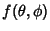expressed as a double sum of Spherical Harmonics is called a Laplace series. Taking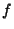as a Complex Function,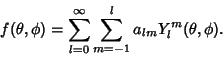(1)

Now multiply both sides by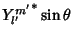and integrate over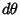and.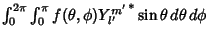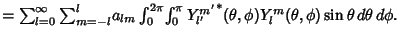(2)
Now use the Orthogonality of the Spherical Harmonics(3)

so (2) becomes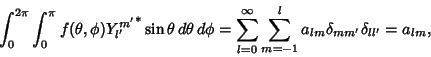(4)

where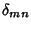is the Kronecker Delta.

For a Real series, consider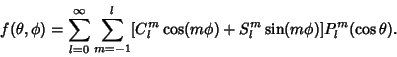(5)

Proceed as before, using the orthogonality relationships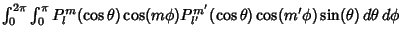(6)(7)
Soand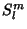are given by(8)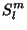(9)